# Construction of 12 DOFs spur gear coupling dynamic model

## Huanchao Lv1, Zhengminqing Li2, Wenlin Zhu3, Xin Tang4, Jie Gao5, Rupeng Zhu6

1, 2, 6National Key Laboratory of Science and Technology on Helicopter Transmission, Nanjing University of Aeronautics and Astronautics, Nanjing, 210016, China

3, 4, 5Science and Technology on Helicopter Transmission Laboratory, AECC Hunan Aviation Powerplant Research Institute, Zhuzhou, 412002, China

2Corresponding author

Vibroengineering PROCEDIA, Vol. 28, 2019, p. 241-245. https://doi.org/10.21595/vp.2019.21028
Received 17 September 2019; accepted 1 October 2019; published 19 October 2019

42nd International Conference on Vibroengineering in Shanghai, China, October 19-21, 2019

Copyright © 2019 Huanchao Lv, et al. This is an open access article distributed under the Creative Commons Attribution License, which permits unrestricted use, distribution, and reproduction in any medium, provided the original work is properly cited.
Views 39
Abstract.

A 12-degree-of-freedom (DOF) spur gear dynamic model is constructed, which is coupled by the mesh gear pair and the gearbox. The construction method of spur gear coupling dynamic model, based on lumped mass method, is better than finite element method, due to higher modeling efficiency. The work would be benefit to spur gear coupling dynamic modeling and analyses.

Highlights
• A dynamic model coupled by the mesh gear pair and the gearbox is constructed.
• The construction method of spur gear coupling dynamic model is more efficient.
• Using modal assurance criterion to verify the accuracy of the proposed method.

Keywords: spur gear, coupling dynamic model, 12 DOFs.

#### 1. Introduction

Gear dynamic models are focused by many scholars. There is an extensive body of literatures on it [1-9]. Jin et al. established gear dynamic models coupled with bending-torsion-axis-swing of mesh pairs based on lumped mass method . Zhu et al. constructed finite element models of the gear transmission, and evaluated dynamic behavior of the system [11, 12]. Ren et al. proposed a construction method of gear dynamic models based on substructure method [13-15]. However, the gear coupling dynamic models associated with mesh pairs and gearbox supports are few studied. Thus, in the paper, a 12 DOFs spur gear coupling dynamic model, based on lumped mass method, is proposed. The work would be helpful to the spur gear coupling dynamic analyses.

#### 2. Construction of 12DOFs dynamic model

The gear transmission system is mainly composed of two spur gears, bearings and gearbox supports. When modeling with the finite element method, it is inefficient because of the complexity of the gearbox supports. Therefore, a 12 DOFs coupling dynamic model based on lumped mass method is established, as shown in Fig. 1.

Fig. 1. The coupling dynamic model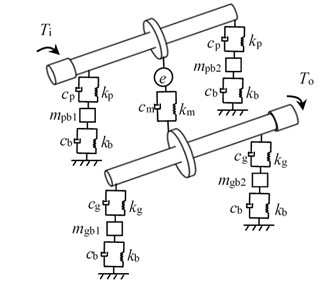As illustrated in Fig. 1, subscript $p$ and $g$ express driving gear and driven gear, respectively, $k$ is a bending stiffness, $c$ is a bending damping, ${k}_{m}$ is a mesh stiffness, ${c}_{m}$ is a mesh damping, $e$ is a static transmission errors (STE), ${T}_{i}$ is the input torsion, ${T}_{o}$ is the output torsion, ${k}_{b}$ is the support stiffness, ${c}_{b}$ is the support damping. Moreover, ${m}_{pb1}$, ${m}_{pb2}$, ${m}_{gb1}$ and ${m}_{gb2}$ are the equivalent masses of the gearbox supports.

As given in Fig. 1, the mathematical equations of the meshing pair could be derived by:

(1)
$\left\{\begin{array}{l}{m}_{p}{\stackrel{¨}{l}}_{p}+{c}_{p}{\stackrel{˙}{l}}_{p}+{k}_{p}{l}_{p}-{F}_{m}=0,\\ {m}_{g}{\stackrel{¨}{l}}_{g}+{c}_{g}{\stackrel{˙}{l}}_{g}+{k}_{g}{l}_{g}+{F}_{m}=0,\\ {I}_{i}{\stackrel{¨}{\theta }}_{i}+{c}_{1}\left({\stackrel{˙}{\theta }}_{i}-{\stackrel{˙}{\theta }}_{p}\right)+{k}_{1}\left({\theta }_{i}-{\theta }_{p}\right)={T}_{i},\\ {I}_{p}{\stackrel{˙}{\stackrel{¨}{\theta }}}_{p}-{c}_{1}\left({\stackrel{˙}{\theta }}_{i}-{\stackrel{˙}{\theta }}_{p}\right)-{k}_{1}\left({\theta }_{i}-{\theta }_{p}\right)-{r}_{p}\cdot {F}_{m}=0,\\ {I}_{g}{\stackrel{¨}{\theta }}_{g}+{c}_{2}\left({\stackrel{˙}{\theta }}_{g}-{\stackrel{˙}{\theta }}_{o}\right)+{k}_{2}\left({\theta }_{g}-{\theta }_{o}\right)+{r}_{g}\cdot {F}_{m}=0,\\ {I}_{o}{\stackrel{¨}{\theta }}_{o}-{c}_{2}\left({\stackrel{˙}{\theta }}_{g}-{\stackrel{˙}{\theta }}_{o}\right)-{k}_{2}\left({\theta }_{g}-{\theta }_{o}\right)=-{T}_{o},\end{array}\right\$

where subscript $i$ and $o$ express motor and load, respectively, $\theta$ is a torsion degree, $l$ is a bending degree, $m$ is a mass, $r$ is a base circle radius, $I$ is a moment of inertia, ${k}_{1}$ and ${k}_{2}$ are torsional stiffness of the shaft, ${c}_{1}$ and ${c}_{2}$ are torsional damping of the shaft, and ${F}_{m}$ could be deduced as:

(2)
${F}_{m}={k}_{m}\cdot \left({r}_{g}{\theta }_{g}-{r}_{p}{\theta }_{p}+e+{l}_{g}-{l}_{p}\right)+{c}_{m}\cdot \left({r}_{g}{\stackrel{˙}{\theta }}_{g}-{r}_{p}{\stackrel{˙}{\theta }}_{p}+\stackrel{˙}{e}+{\stackrel{˙}{l}}_{g}-{\stackrel{˙}{l}}_{p}\right).$

The gearbox supports dynamic equivalent model is proposed, as shown in Fig. 2.

Fig. 2. The gearbox supports dynamic equivalent model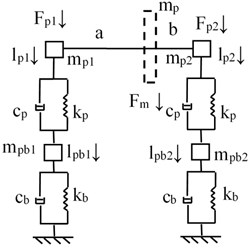a) Equivalent model of the driving gear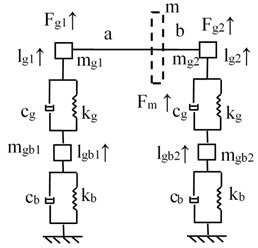b) Equivalent model of the driven gear

As illustrated in Fig. 2, the equivalent mass of the gears at the bearing fulcrum could be deduced as:

(3)
$\left\{\begin{array}{l}{m}_{p1}={m}_{p}\cdot \frac{b}{a+b},\\ {m}_{p2}={m}_{p}\cdot \frac{a}{a+b},\\ {m}_{g1}={m}_{g}\cdot \frac{b}{a+b},\\ {m}_{g2}={m}_{g}\cdot \frac{a}{a+b},\end{array}\right\$

where $a$ and $b$ are the distance from the gear to the bearing fulcrum.

As given in Fig. 2, the mathematical equations of the support structure could be derived by:

(4)
$\left\{\begin{array}{l}{m}_{p1}{\stackrel{¨}{l}}_{p1}+{c}_{p}\left({\stackrel{˙}{l}}_{p1}-{\stackrel{˙}{l}}_{pb1}\right)+{k}_{p}\left({l}_{p1}-{l}_{pb1}\right)-{F}_{p1}=0,\\ {m}_{pb1}{\stackrel{¨}{l}}_{pb1}+{c}_{b}{\stackrel{˙}{l}}_{pb1}+{k}_{b}{l}_{pb1}-{c}_{p}\left({\stackrel{˙}{l}}_{p1}-{\stackrel{˙}{l}}_{pb1}\right)-{k}_{p}\left({l}_{p1}-{l}_{pb1}\right)=0,\\ {m}_{g1}{\stackrel{¨}{l}}_{g1}+{c}_{g}\left({\stackrel{˙}{l}}_{g1}-{\stackrel{˙}{l}}_{gb1}\right)+{k}_{g}\left({l}_{g1}-{l}_{gb1}\right)-{F}_{g1}=0,\\ {m}_{gb1}{\stackrel{¨}{l}}_{gb1}+{c}_{b}{\stackrel{˙}{l}}_{gb1}+{k}_{b}{l}_{gb1}-{c}_{g}\left({\stackrel{˙}{l}}_{g1}-{\stackrel{˙}{l}}_{gb1}\right)-{k}_{g}\left({l}_{g1}-{l}_{gb1}\right)=0,\\ {m}_{p2}{\stackrel{¨}{l}}_{p2}+{c}_{p}\left({\stackrel{˙}{l}}_{p2}-{\stackrel{˙}{l}}_{pb2}\right)+{k}_{p}\left({l}_{p2}-{l}_{pb2}\right)-{F}_{p2}=0,\\ {m}_{pb2}{\stackrel{¨}{l}}_{pb2}+{c}_{b}{\stackrel{˙}{l}}_{pb2}+{k}_{b}{l}_{pb2}-{c}_{p}\left({\stackrel{˙}{l}}_{p2}-{\stackrel{˙}{l}}_{pb2}\right)-{k}_{p}\left({l}_{p2}-{l}_{pb2}\right)=0,\\ {m}_{g2}{\stackrel{¨}{l}}_{g2}+{c}_{g}\left({\stackrel{˙}{l}}_{g2}-{\stackrel{˙}{l}}_{gb2}\right)+{k}_{g}\left({l}_{g2}-{l}_{gb2}\right)-{F}_{g2}=0,\\ {m}_{gb2}{\stackrel{¨}{l}}_{gb2}+{c}_{b}{\stackrel{˙}{l}}_{gb2}+{k}_{b}{l}_{gb2}-{c}_{g}\left({\stackrel{˙}{l}}_{g2}-{\stackrel{˙}{l}}_{gb2}\right)-{k}_{g}\left({l}_{g2}-{l}_{gb2}\right)=0.\end{array}\right\$

According to the deformation coordination relationship, as shown in Fig. 3, the deformation coordination equations could be derived by:

(5)
$\left\{\begin{array}{l}{l}_{p}=\frac{b{l}_{p1}+a{l}_{p2}}{a+b},\\ {l}_{g}=\frac{b{l}_{g1}+a{l}_{g2}}{a+b}.\end{array}\right\$

Fig. 3. The coordination relationship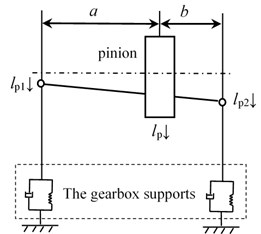According to the deformation coordination Eq. (5), Eq. (1) and Eq. (4), a 12 DOFs coupling dynamic model, based on lumped mass method, is established.

#### 3. Simulations

In order to verify the accuracy of the proposed method, the parameters of an example case are listed in Table 1.

Table 1. Parameters of system

 Symbol name Value Unit Modulus / $m$ 4 mm Pressure angle / $\alpha$ 20 ° Tooth number of driving gear / ${z}_{1}$ 23 – Tooth number of driven gear / ${z}_{2}$ 69 – Addendum coefficient / ${h}_{a}^{*}$ 1 – Clearance coefficient / ${c}^{*}$ 0.25 –

According to the 12 DOFs coupling dynamic model and the parameters listed in Table 1, the natural frequencies of the example case are simulated. Part of the results are shown in Fig. 4.

In the case of Fig. 4, the natural vibration mode vector of the first-order non-zero natural frequency (second frequency: 1403 Hz) is:

(6)

Fig. 4. Natural frequency simulations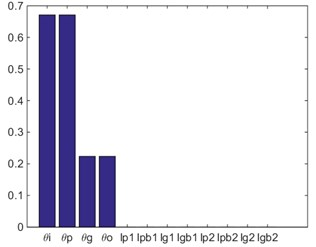a) First frequency: 0 Hz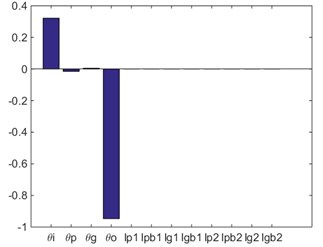b) Second frequency: 1403 Hz

According to the simulation result based on finite element model, as shown in Fig. 5, the natural vibration mode vector could be expressed as:

(7)

Fig. 5. Natural vibration mode based on FEM (natural frequency: 1282.9 Hz)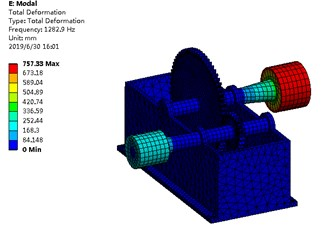According to the modal assurance criterion (MAC), Eq. (6) and Eq. (7), the natural vibration mode vector correlation can be derived by:

(8)
$MAC=\frac{{\left|{\varphi }_{B}^{T}{\varphi }_{A}\right|}^{2}}{{\varphi }_{B}^{T}{\varphi }_{B}{\varphi }_{A}^{T}{\varphi }_{A}}.$

According to Eq. (8), the MAC value of the example case is 0.9997, namely, the natural vibration mode shown in Fig. 4(b) and the natural vibration mode shown in Fig. 5 are the same-order physical mode. The relative error of the natural frequencies between two methods is calculated, as shown in Table 2.

Table 2. The relative error of the natural frequencies between two methods

 Value Unit The natural frequency based on lumped mass method 1403 Hz The natural frequency based on FEM 1282.9 The relative error 9.36 %

In the case of Table 2, the relative error of the natural frequencies between two methods is 9.36 %, namely, the proposed method is accurate and feasible.

#### 4. Conclusions

In the issue, a 12 DOFs spur gear coupling dynamic model, based on lumped mass method, is proposed. The construction method of spur gear coupling dynamic model is better than finite element method, because it enables rapid modeling of complex gearbox and makes dynamic modeling more efficient. This contribution would be helpful to the spur gear coupling dynamic modeling and analyses.

#### Acknowledgements

The authors are grateful for the financial support provided by the National Natural Science Foundation of China under No. 51775264, and by National Key Laboratory of Science and Technology on Helicopter Transmission (Nanjing University of Aeronautics and Astronautics) (Grant No. HTL-A-19G06). In addition, the authors declare that there is no conflict of interests regarding the publication of this article.

1. Mark W. D. Use of the generalized transmission error in the equations of motion of gear systems. Journal of Mechanical Design, Vol. 109, Issue 2, 1987, p. 283-291. [Publisher]
2. Şener Ö. S., Özgüven H. N. Dynamic analysis of geared shaft systems by using a continuous system model. Journal of Sound and Vibration, Vol. 166, Issue 3, 1993, p. 539-556. [Publisher]
3. Walha L., Louati J., Fakhfakh T., et al. Dynamic Response of Two-Stage Gear System Damaged by Teeth Defects. Oficyna Wydawnicza Politechniki Warszawskiej, 2005. [Search CrossRef]
4. Bodas A., Kahraman A. Guda-1 influence of carrier and gear manufacturing errors on the static planet load sharing behavior of planetary gear sets (gear unit design and applications). The Japan Society of Mechanical Engineers, 2017, p. 633-638. [Publisher]
5. Li Z., Zhu R. Sensitivity predictions of geometric parameters on engagement impacts of face gear drives. Journal of Vibroengineering, Vol. 17, Issue 5, 2015, p. 2236-2246. [Search CrossRef]
6. Hu Z. H., Tang J. Y., Chen S. Y., Lei D. C. Effect of mesh stiffness on the dynamic response of face gear transmission system. Journal of Mechanical Design, Vol. 135, Issue 7, 2013, p. 071005. [Publisher]
7. Fernandez A., Garcia P., Ibarbia A. D. Enhanced model of gear transmission dynamics for condition monitoring applications: Effects of torque, friction and bearing clearance. Mechanical Systems and Signal Processing, Vol. 85, 2017, p. 445-467. [Publisher]
8. Rigaud E., Sabot Effect J. Elasticity of shafts, bearings, casing and couplings on the critical rotational speeds of a gearbox. Physics, Vol. 1230, 2007, p. 833-845. [Search CrossRef]
9. Shaobin L. Study on Coupled Thermo-Elastic Deformation and Nonlinear Dynamic Emulate about High-Speed, Heavy-Load Gear Transmission System. Chongqing University, 2004. [Search CrossRef]
10. Jin G., Zhu R., Bao H. Nonlinear dynamical characteristics of face gear transmission system. Journal of Central South University, Vol. 5, Issue 41, 2010, p. 1807-1813. [Search CrossRef]
11. Zhu C. C., Lu B., Xu X. Y., et al. Analysis of coupling characteristics of transmission system and structural system of high power marine gearbox. Journal of Ship Mechanics, Vol. 15, Issue 11, 2011, p. 1315-1321. [Search CrossRef]
12. Zhu C. C., Lu B., Xu X. Y., et al. Analysis of heavy duty marine gearbox with thermal-elastic coupling. Journal of Ship Mechanics, Vol. 15, Issue 8, 2011, p. 898-905. [Search CrossRef]
13. Ren Y. F., Chang S., Liu G., et al. Analysis of influence of box flexibility on dynamic characteristics of gear transmission system. Journal of Vibration and Shock, Vol. 14, 2017. [Publisher]
14. Liu L., Zhao C. Q., Ren Y. F., et al. Dynamic modeling and box influence analysis of gearbox fully coupled system. Journal of Harbin Engineering University, Vol. 39, Issue 3, 2018, p. 561-568. [Search CrossRef]
15. He Z. X., Zhao C. Q., Ren Y. F., et al. Dynamic response analysis of planetary gear transmission system with coupling box vibration. Journal of South China University of Technology, Vol. 9, 2015, p. 128-134. [Search CrossRef]

#### Cited By

 Computational Intelligence and Neuroscience Song Ding, Jun Ye 2022 Applied Sciences Haiwei Wang, Cheng Ji, Fengxia Lu, Cheng Wang, Xueyan Sun 2022